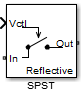# SPST

Single pole single throw switch

## Library

Junctions

•## Description

The SPST block models a single pole single throw switch. The input Simulink® signal, Vctl, controls when the signal at the input port is transferred to the output port.

For resistance characterization:

• If the control voltage is less than the threshold voltage, the block attenuates the signal using the resistance value specified in Off resistance. The block places the resistor between the positive input and output terminals.

• If the control voltage is greater than or equal to the threshold voltage, the block attenuates the signal using the resistance value specified in On resistance.

• When the switch loading type is absorptive, and the control voltage is less than the threshold voltage, the block places internal shunt resistors at the input and output ports.

For insertion loss characterization:

• If the control voltage is less than the threshold voltage, calculate the off resistance value using the Isolation (dB) value.

• If the control voltage is greater than or equal to the threshold voltage, calculate the on resistance value using the Insertion loss (dB) value.

• When the switch loading type is absorptive, and the control voltage is less than the threshold voltage, the block places internal shunt resistors at the input and output ports.

The shunt resistor values provide matching terminations at the input and output ports.

The voltage–current relationship at the terminals depends on the relationship between control voltage, Vctl, and the threshold voltage, Vthreshold.

• If Vctl < Vthreshold

`$\begin{array}{l}{I}_{in}\text{\hspace{0.17em}}\text{\hspace{0.17em}}\text{ }\text{ }={V}_{inout}.{G}_{off}^{}+{V}_{in}.{G}_{Z01}\\ {I}_{out}=-{V}_{inout}.{G}_{off}+{V}_{out}.{G}_{Z02}\end{array}$`
• If VctlVthreshold

`$\begin{array}{l}{I}_{in}={V}_{inout}.{G}_{off}^{}\\ {I}_{out}\text{\hspace{0.17em}}\text{\hspace{0.17em}}\text{ }\text{ }=-{V}_{inout}.{G}_{on}\end{array}$`

• Gon, Goff — On and off path conductances

• GZ01, GZ02 — Shunt port conductances

## Parameters

Threshold voltage

Threshold voltage of the switch, specified as a positive scalar. The default value is `0`  volts.

Characterization

Characterization of the `SPST` switch: `Resistance` or ```Insertion loss```. The default value is `Resistance`.

On resistance

On resistance value of the switch, specified as a positive scalar. The default value is `10`  ohms. On resistance is available when you set Characterization to `Resistance`.

Off resistance

Off resistance value of the switch, specified as a positive scalar. The default value is `1e6`  ohms. Off resistance is available when you set Characterization to `Resistance`.

Insertion loss (dB)

Insertion loss value of the switch, specified as a positive scalar. The default value is `1`  dB. Insertion loss (dB) is available when you set Characterization to ```Insertion loss```.

Isolation (dB)

Isolation value of the switch, specified as a positive scalar. The default value is `70`  dB. Isolation (dB) is available when you set Characterization to `Insertion loss`

Loading type of the `SPST` switch: `Absorptive` or `Reflective`. The default is `Reflective`.

Port terminations

Port termination that matches the impedance conditions of the `SPST` switch. The default value is `50`  ohms.

### Note

Port terminations is available when you set the `SPST` switch Characterization to `Insertion loss` or when you set Loading type to `Absorptive`.

Ground and hide reference terminals

Select this option to internally ground and hide the reference terminals. Clear the option to expose the reference terminals. By exposing these terminals, you can connect them to other parts of your model.

By default, this option is selected.